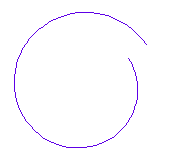# Class Reference pointArray

An array of points to store polygon or path data. More...

### Member

 pointArray( ) pointArray(pointArray array) pointArray(int size) void addCornerArea(double value1,double value2,int angleInt=0) void append(point p) void attach(int x, int y) void attachPoint(point p) pointArray bezier2(point pstart, point pend, point pbezier,int maxDepth=-2) pointArray bezier3(point pstart, point pend, point pbezierstart, point pbezierend, int maxDepth=-2) pointArray BSpline() point center() point centerOfGravity() void chamfer(double value,int type,int angleInt=0) pointArray circle(point center,int radius,double angelstep) void clear() pointArray coil(point center,point p1,point p2,int turns, double angelstep) pointArray ellipse(point center,int rx,int ry,double angelstep) void filletBezier(double value,int type,int angleInt=0) void filletRound(double value,int type,int angleInt=0) void flip() void insert(uint i, point p) point maximum() point minimum() point point(int i) void resize(int size) void set(int i, int x, int y) void setPoint(int i,point p) int size() pointArray spirale(point center,point p1,point p2,double angelstep)

### Detailed Description

This class stores an array of class point. The size of the array can be adjusted at runtime and has no limitation. It can be used to create paths, polygons or other shapes.

Example:

``````
pointArray pa=l->thisElement->getPoints();
string sx,sy;
for (i=0; i<pa.size(); i++){
p=pa.point(i);
sx.setNum(p.x());
sy.setNum(p.y());
}``````

### Member Function Documentation

##### pointArray::pointArray( )

Creates an empty array of points.

##### pointArray::pointArray(pointArray array)

Creates an array of points containing a copy of array

##### pointArray::pointArray(int size)

Creates a array of points with the given size.

##### void pointArray::addCornerArea(double value1,double value2,int angleInt=0)

Modifies a pointArray in the same way as cell::addCornerAreaSelect it does for selected polygons.

##### void pointArray::append(point p)

Add the point p to the end of the pointArray. The size of the array will be increased. This method is identical with attachPoint and was introduced with release 20210215 to get an identcal syntax with other list classes.

##### void pointArray::attach(int x, int y)

Add a point with the cooridinates (x,y) to the end of the pointArray. The size of the array will be increased.

##### void pointArray::attachPoint(point p)

Add the point p to the end of the pointArray. The size of the array will be increased.

##### static pointArray pointArray::bezier2(point pstart, point pend, point pbezier,int maxDepth=-2)

Returns: a pointArray with the shape of a bezier curve in second order. maxDepth will set the iterations. An increase of one will double the number of points. A value of -2 will use the defaults from the setup. (introduced with release 20190807)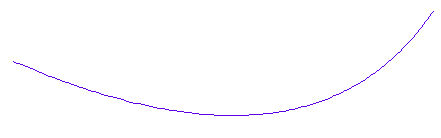##### static pointArray pointArray::bezier3(point pstart, point pend, point pbezierstart, point pbezierend, int maxDepth=-2)

Returns: a pointArray with the shape of a bezier curve in third order. maxDepth will set the iterations. An increase of one will double the number of points. A value of -2 will use the defaults from the setup. (introduced with release 20190807)##### pointArray pointArray::BSpline()

a B-Spline is created and returned, if the first point is identical with the last point a closed B-Spline is assumed. (introduced with release 20190202)open and closed B-Spline, red entered pointArray, blue resulting path/polygon

##### point pointArray::center()

Returns: the center of of the bounding box of the polygon (introduced with release 20180623)

##### point pointArray::centerOfGravity()

Returns: the center of gravity of the polygon

##### void pointArray::chamfer(double value,int type,int angleInt=0)

Modifies a pointArray in the same way as cell::chamferSelect it does for selected polygons.

Option Result
type 0
chamfer length is always the same value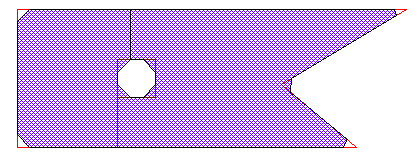type 1
chmafer size differ on angle
resulting length of edge is the same value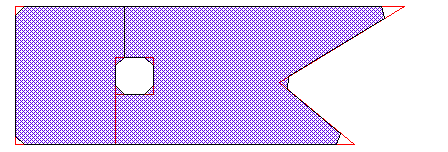##### static pointArray pointArray::circle(point center,int radius,double angelstep)

Returns: a pointArray with the shape of a circle. angelstep will set the resolution in deg/point. In case zerro is entered the default circle resultion from the setup is used. (introduced with release 20190807)

##### void pointArray::clear()

Removes all items from the list. (introduced with release 20230121)

##### static pointArray pointArray::coil(point center,point p1,point p2,int turns, double angelstep)

Returns: a pointArray with the shape of a coil from p1 to p2. angelstep will set the resolution in deg/point. In case zerro is entered the default circle resultion from the setup is used. (introduced with release 20211010)##### static pointArray pointArray::ellipse(point center,int rx,int ry,double angelstep)

Returns: a pointArray with the shape of a ellipse. rx and ry will set he radius in the x- and y-axis. angelstep will set the resolution in deg/point. In case zerro is entered the default circle resultion from the setup is used. (introduced with release 20190807)

##### void pointArray::filletBezier(double value,int type,int angleInt=0)

Modifies a pointArray in the same way as cell::filletBezierSelect it does for selected polygons. Corners are replaced by Bezier curves. type will influence the size in the same way chamfer does.##### void pointArray::filletRound(double value,int type,int angleInt=0)

Modifies a pointArray in the same way as cell::filletRoundSelect it does for selected polygons. Corners are replaced by circular curves. type will influence the size in the same way chamfer does.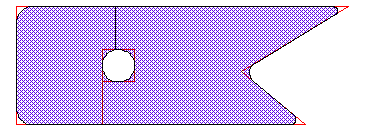##### void pointArray::flip()

will flip the order of the point in the array. First point will get last point, etc.

##### void pointArray::insert(uint i, point p)

Inserts the point p at position i into the array. The size of the array will be increased.

##### point pointArray::maximum()

Returns: the upper right corner of the bounding box of the polygon (introduced with release 20180623)

##### point pointArray::minimum()

Returns: the lower left corner of the bounding box of the polygon (introduced with release 20180623)

##### point pointArray::point(int i)

Returns: the point at the position i

##### void pointArray::resize(int size)

Changed the size of the array. If size is bigger than the current size of the array, new points will be added to the end of the array. If it is smaller, points at the end will be deleted.

##### void pointArray::set(int i, int x, int y)

Changed the coordinates of the point at position i to the values x and y.

##### void pointArray::setPoint(int i,point p)

Sets the point p at the index of i.

##### int pointArray::size()

Returns: the size of the array

##### static pointArray pointArray::spirale(point center,point p1,point p2,double angelstep)

Returns: a pointArray with the shape of a spiral from p1 to p2. angelstep will set the resolution in deg/point. In case zerro is entered the default circle resultion from the setup is used. The spiral has always a single turn. For multi turn spiral please use coil instead. (introduced with release 20190807)